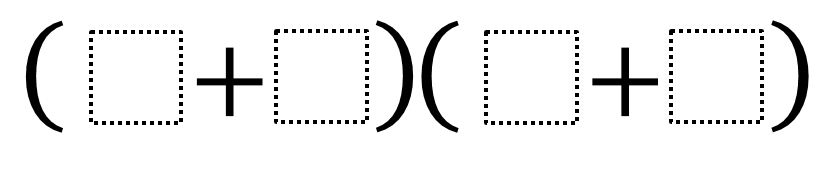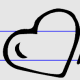# Biggest Product

Directions: Using the digits 1 to 9 at most one time each, fill in the boxes to make the biggest/smallest product.### Hint

In order to make the biggest/smallest product, which numbers should we use?

Biggest Product: (9+6)(8+7)
Smallest Product: (1+2)(3+4)

Source: Nanette Johnson

## Product Close to 1,000

Directions: Using the digits 1 to 9 at most one time each, place a digit …

1.when it says biggest/smallest I assumed there would be an answer for the biggest and the smallest

•Thank you for pointing that out. We will add the smallest product…how did you get your answer for the smallest? Did you get a smaller answer? =)

2.(5+4) (6+4)

3.(1+2)+(3+4)

4.(2+4)(6+8)

5.sorry I meant (1+2)(3+4)

6.(8+5)(4+5)

7.(1*8)(2*4)

8.(5+7)(8+6)=
12×14=168

9.(1+9)(2+8)
(1+2)(2+0)

10.(9+6)(8+7)

(1+2)(3+4)

11.12.(9+6) + (8+7) = 30
(1+2) + (3+4) =10